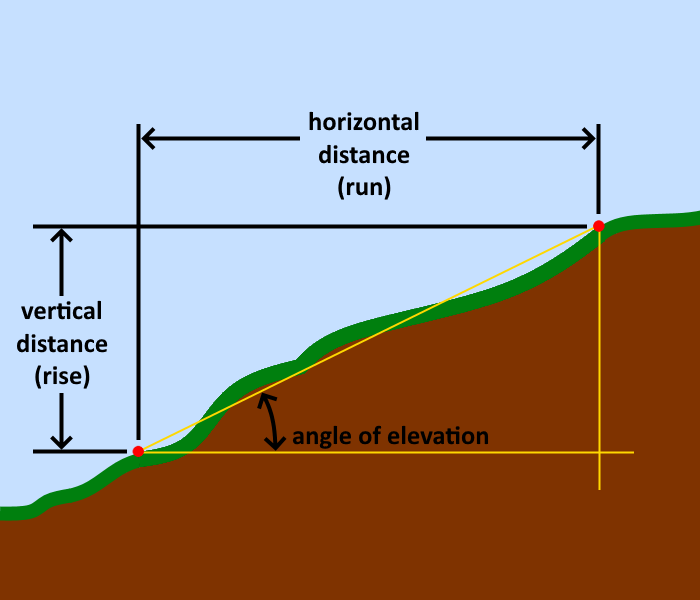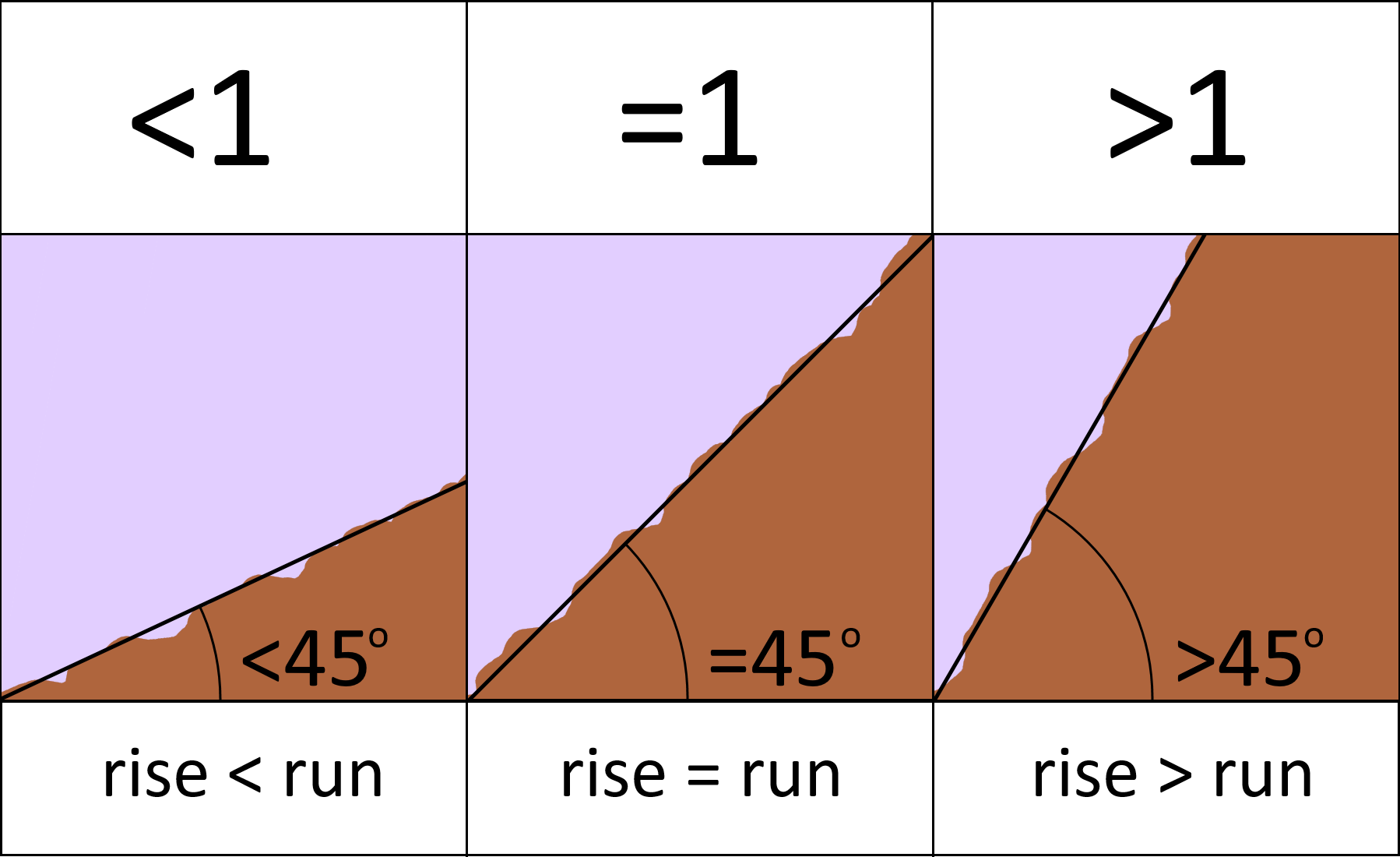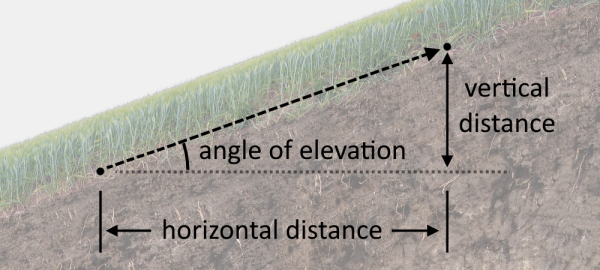Created by Kenneth Alambra
Reviewed by Dominik Czernia, PhD and Jack Bowater
Last updated: Feb 02, 2023

This elevation grade calculator determines and expresses, in four different ways, the slope of an earthen surface. It may be given in terms of the angle of elevation from the horizontal plane, in terms of the percentage of deviation from the horizontal line, or simply in terms of the ratio of its "rise to run" or "unit rise in run". To calculate the slope of a line and to learn more about slopes in general, you can check our slope calculator. To learn more about how to find the slope of the terrain (or of the ground in general), keep reading.Elevation grade (or slope) is the steepness, or degree of inclination, of a certain area of land. It can simply be the steepness between two specific points in a given area, the average of an area's gradual change in steepness, or an erratic variation in the elevation of the ground. We usually measure the ground's elevation as its altitude above sea level. Land surveyors and engineers are generally the ones who measure and record these elevations using surveying equipment and tools.

## Why do we need to know a particular terrain's elevation grade?Knowing an area's elevation grade offers many advantages over not knowing it at all. It may also be a requirement to proceed with other activities, such as construction or irrigation. Here are some examples of the importance and advantages of knowing the grade of a particular area:

• Knowing the area's elevation grade helps in the construction of roads. We use it, for example, in the vertical curve formula. Very steep terrain may require roads to be laid out in a zig-zag pattern for safer ascending and descending. Engineers can design a cutting-and-filling procedure for less steep terrain to decrease the slope. Cutting-and-filling is where soil from the higher part of the slope is transferred to the lower part to reduce its grade.
• Determining the elevation grade also helps when building retaining walls. Retaining walls support the earth above it to keep it from eroding downhill. Therefore a retaining wall can be constructed against a cliff to avoid landslides.
If you wish to learn more about retaining walls, retaining walls check out our retaining wall calculator.
• Knowing the terrain's angle of elevation also helps when assessing a land's ability to support a structure. Building structures on a sloping area may require some additional foundation to avoid failing.
• Elevation grade also helps when examining the flow of water from higher elevations to see if the land can be irrigated without pumps.
• and so much more. :)

## How to calculate for the elevation grade and angle of elevation?

We calculate terrain grade in the same way we calculate the slope of a line. The slope of a given line requires the coordinates of its endpoints. The slope is the quotient of the change in the values of y and the change in the values of x. As an equation, it looks like this:

$\footnotesize \text{slope} = \frac{y_2 - y_1}{x_2 - x_1}$

In the mathematical community, these changes in x and y values have nicknames. We call the change in the values of y "rise", since it is along the vertical axis. On the other hand, we call the change in the x values the "run", since it runs along the horizontal axis. This relationship gives us the popular equation that you might have already heard before: "slope is equal to rise over run." Or:

$\footnotesize \text{slope} = \frac{\text{rise}}{\text{run}}$

Aside from this, we can also express grade in terms of angles. With this, we would need the help of trigonometry to obtain the angle. We are interested in getting the angle between the horizontal plane and the plane of incline. We call an angle that goes up from the horizontal line an "angle of elevation", while those that go down are the "angle of declination." We can determine this angle using the arctan function. The arctan function is the inverse of the tangent function with the slope as its article. In equation form, it looks like this:

$\footnotesize \text{Grade (angle)} = \arctan{\frac{\text{rise}}{\text{run}}}$

If you don't have a calculator with this trigonometric function, don't worry, we already have it incorporated into our elevation grade calculator above. If you want to learn more about the other essential trigonometric functions, you can check our trigonometric functions calculator and play around with angles.

Aside from expressing grade using ratios and angles, we can also determine it in terms of percentages - we only have to multiply its ratio value by 100 to get it. That is where the equation below comes from. A grade of one hundred percent means that the slope's rise equals its run. The resulting slope for this value is considered neither steep nor flat but instead of an average grade. Here is its equation form:

$\footnotesize \text{Grade (percentage)} = \frac{\text{rise}}{\text{run}} \cdot 100\%$

We equipped our elevation grade calculator with all these equations, so it can give you all the answers you need every time you input a value for any variable. You can set the grade and determine the horizontal distance required to obtain the required change in a vertical distance, as well as obtain the angle of elevation.

Grade values are pretty easy to understand. Terrain grades less than 1 (terrain grade <1) mean the slope is gentle. On the other hand, terrain grades that are greater than one (terrain grade >1) indicate that the terrain is steep. In terms of angle of elevation, angles above 45 degrees are the steep ones, whereas angles below 45 degrees are relatively flat.## How to determine the "rise" and "run."

We can get the "run", or the change in the horizontal distance, by using a measuring tape. We will have to pull the tape taut to get a precise measurement. However - not pulling it tight will provide a higher value than this actual value because the tape will sag due to gravity.

On the other hand, we can get the vertical distance by using optical devices, such as a surveyor's transit paired with a leveling rod. A surveyor's transit has an inbuilt telescope that can be rotated laterally or vertically over a tripod. Focusing it on the leveling rod (which is like a huge ruler) will give a reading. We can translate this reading into the elevation between the leveling rod and the transit. A surveyor's transit also has distinctive markings on its view that make it also able to measure horizontal distances when paired with a leveling rod.We can also use a tool like a clinometer, which can directly provide the angle of elevation between two points. A clinometer is like a mini telescope with a protractor attached to its side. Other more sophisticated digital gadgets can now help obtain the slope of a particular surface. A smartphone, if equipped with adequate hardware like gyroscopic sensors, can accurately provide you with a surface's slope when you lay your it on the slope.

We can plot the averages of the terrain grades to compose a contour map. A contour map is a topographic map that gives viewers an overview of what a specific area's terrain is like. Vertical exaggeration is often needed to emphasize certain terrain features, such as the height of the mountains or volcanoes.

## FAQ

### What's the elevation grade of the steepest road in the world?

Baldwin Street, New Zealand, has an impressive grade of about 0.35, corresponding to a horizontal length of 2.86 m for each vertical meter. The short 350 m long street is a real challenge to walk up!

A grade of 0.35 correspond to an angle of:
angle = arctan(rise/run) = arctan(1/2.86) = 19.27°.

### What's the difference between slope as an angle and slope as a percentage?

The formula gives the slope defined as an angle:
slope (angle) = arctan(rise/run)
Where the rise is the vertical distance and the run is the horizontal distance of a slope.
To calculate the slope as a percentage, take the argument of the arctangent function and multiply it by 100:
slope (percentage) = rise/run × 100

### Which angle corresponds to a slope of 100%?

Rather counterintuitively, a slope of 100% corresponds to an angle of 45°. However, consider that the slope is the result of the equation:
slope = rise/run
Where

• rise is the vertical distance; and
• run is the horizontal one.

A slope where each horizontal meter corresponds to a vertical meter has slope 1, which multiplied by 100 returns the value 100%.

Let's assume that the road has 12 meters of rise for every 200 meters of run. The grade would be 0.06. To calculate the grade of a road with:

• rise = 12 m; and
• run = 200 m:
1. Compute the ratio between rise and run:
grade = rise/run = 12/200 = 0.06
2. If you want to know the angle of the slope, input the value in the arctangent function:
slope (angle) = arctan(rise/run) = arctan(12/200) = 3.43°
3. If you want to know the slope as a percentage, multiply the ratio by 100:
slope (percentage) = 100 × rise/run = 6%.
Kenneth AlambraVertical distance ("rise")
ft
Horizontal distance ("run")
ft
%
Angle of elevation
You might also see this elevation grade expressed in the ratio of 1 in 20. 🙂
People also viewed…

### Concrete weight

Use the concrete weight calculator to see how much a specific volume of concrete weighs.

### Glass weight

Find the weight of any volume of glass material made from the most common glass types in production today using this glass weight calculator.

### Meat footprint

Check out the impact meat has on the environment and your health.

### Pizza size

This calculator will help you make the most delicious choice when ordering pizza.